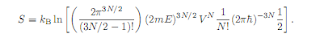## Friday, August 27, 2010For Equation 6.6 Nelson briefly mentions a very important constant: the Planck Constant. The text states vaguely that it comes from quantum mechanics and it is used in the Sakur-Tetrode formula because it has the correct units. Here is the brief but real story.

When a young scientist Max Planck was working on developing a formula for predicting black-body radiation he happened across the concept of quantised energy. He found that heat radiation was produced in multiples of a discrete and very small number. The number is now known as the Planck constant and it has the value of ~6.63 x 10^-34 Js.
Planck derived the equation of E = hv by assuming that radiated heat was produced by atoms oscillating in harmonic motion between discrete energies. The difference between the energies were characterised by the frequency v and the energy constant h. Planck’s work was clear evidence against the previous assumption that energy was a continuous variable. His work led to Einstein’s proposal of light existing as photons as well as Schrodinger’s quantum wave mechanics.
The Planck constant also applies to energies concerning angular frequency. The reduced Planck constant (or h-bar) is equal is h/2π and is used in the equation E = ħω.

For more details see:
Planck Constant Wiki

1.2.